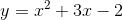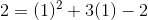Scan QR code or get instant email to install app

Question:

# 1212Solve this system of equations:-6x + 10y = 14 x + 2y = 5

A (1, 2)
explanation

1212The solution is the value or set of values that satisfies each of the equations. Because lines can intersect at only one point, if there is a solution, it must be the point at which the lines intersect. Begin by finding the solution to the system of linear equations. Elimination will be used to solve for the variable x. Multiply the second equation by -5 and combine the two lines to solve for x: (-6x + 10y = 14) + (-5x - 10y = -25), gives -11x = -11, so x = 1. Substitute this value into either linear equation to solve for y: 1 + 2y = 5, so y = 2 To confirm that (1, 2) is a solution, substitute it into the quadratic equation to test if it yields a true statement:, which becomes 2 = 1 + 3 - 2, which gives 2 = 2, so the only solution is (1, 2)